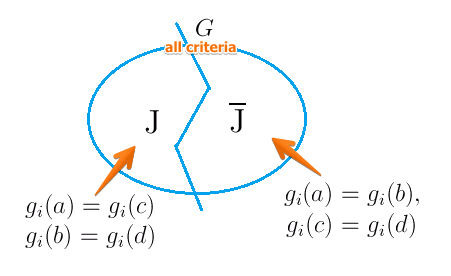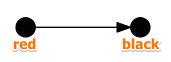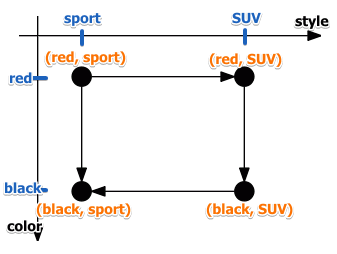# ML Wiki

## Preferential Independence

The preference independence principle is an important principle from MCDA for choosing criteria: they should be preferential independent.

Suppose we have 4 alternatives $a,b,c,d$ and a subset of criteria $J \subset G$ such that

• $g_i(a) = g_i(b), \forall i \not \in J$
• $g_i(c) = g_i(d), \forall i \not \in J$
• $g_i(a) = g_i(a), \forall i \in J$
• $g_i(b) = g_i(d), \forall i \in J$• for criteria that are in $J$, $a$ is the same as $c$ and $b$ is the same as $d$
• for criteria not in $J$, $a$ is the same as $b$, $c$ is the same as $d$

Preferential Independence

• $J \subset A$ is preferentially independent within $G$ when
• if $\forall a,b,c,d \in A$ these conditions hold
• then $a \ P \ b \iff c \ P \ d$

if a decision maker says $a \ P \ b$ we know that he bases his opinion on the set of $J$, because in $\overline{J}$ $a \ I \ b$ - they are the same

## Examples

### Example 1

$g_1$ $g_2$ $g_3$
$a$ 45 70 100
$b$ 50 70 80
$c$ 45 90 100
$d$ 50 90 80

criteria $\{g_1, g_3\}$ are preferentially independent

• i.e. $a \ P \ b \iff c \ P \ d$

### Example 2

In this example the Preferential Independence principle is not satisfied

We're in a restaurant and there are 2 dishes and 2 drinks

• dishes: fish, meat
• drinks: red wine, white wine
• so we have 4 combinations:
drinks $\downarrow$
meal $\to$ $(a)$ fish + white $(c)$ fish + red
$(b)$ meal + white $(d)$ meal + red

So we have two criteria:

• $g_1$ - meal
• $g_2$ - drink

For meal:

• $g_1(a) = g_1(c)$
• $g_1(b) = g_1(d)$

For drink:

• $g_2(a) = g_2(b)$
• $g_2(c) = g_2(d)$

Not satisfied:

• If, when asked "what would you prefer - meat or fish", the decision maker asks "with what drink"
• then the preferential independence is not satisfied: these two criteria are dependent
• usually the case in real life

Satisfied

• if a DM can say
• "I always prefer meat to fish" ($b \ P \ a \land d \ P \ c$) and
• "I always prefer red wine to white wine" ($c \ P \ a \land d \ P \ b$)
• then if he says "I prefer meat with red wine to meat with white line" then he will say "I prefer fish with red wine to fish with white line" ($d \ P \ c \Rightarrow c \ P \ a$)
• usually not the case

### Example 3

Taken from 

Suppose we are choosing a car and there are two criteria

• style: sport, SUV
• color: red, black

Color is preferentially independent from style when

• if the DM prefers:
• (red, sport) to (black, sport)
• (red, SUV) to (black, SUV)
• then the color is preferentially independent from style
• red is preferred to black regardless of style
•However style is not necessarily independent from color

• if DM prefers
• (red, sport) to (red, SUV), but
• (black, SUV) to (black, sport)
• then the style is not preferentially independent from color
• because the color influences what decision maker prefers

With graphical depiction it's clear:

•• red is always preferred to black (all edges come from red to black)
• but when it comes to style, it's not the case: one edge comes from sport to SUV, another from SUV to sport

### Example 4

Consider a case when an employer wants to hire a new worker on the basis of their age, degree and professional experience.

Worker $g_A$: Age $g_D$: Degree $g_E$: Experience
$a$ 25 Master No Experience
$b$ 25 No Degree 3 Years
$c$ 35 Master No Experience
$d$ 35 No Degree 3 Years

We see that given $J = \{g_A\}$ and $\overline{J} = \{ g_D, g_e \}$:

• $g_i(a) = g_i(b), \forall i \not \in J$
• $g_i(c) = g_i(d), \forall i \not \in J$
• $g_i(a) = g_i(a), \forall i \in J$
• $g_i(b) = g_i(d), \forall i \in J$

However an employee would prefer:

• $a \ P \ b$ but $d \ P \ c$